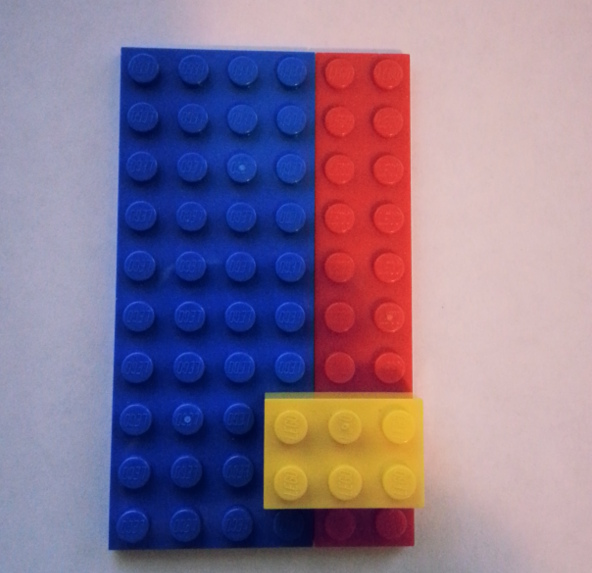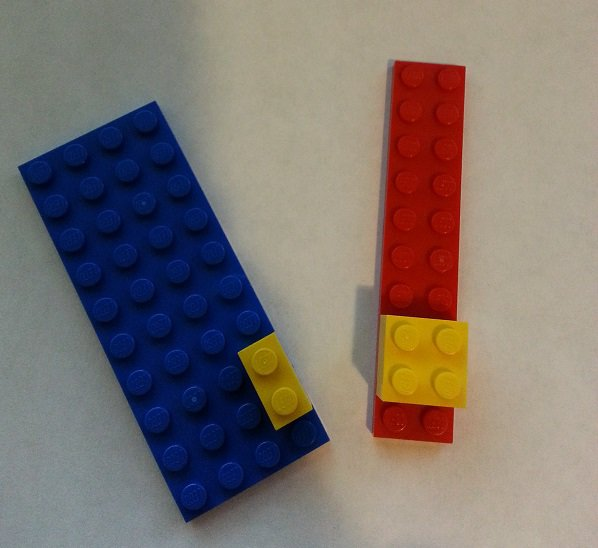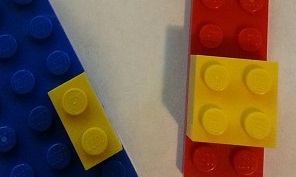# 樂高中的貝氏定理

### 從小小的積木開始### 條件機率P(有黃塊|紅底) = 有黃塊的紅底格數 / 紅底格數 = 4/20 = 1/5。P(紅底|有黃塊) = 有黃塊的紅底格數/有黃塊格數 = 4/6 = 2/3。

### 導出貝氏定理

P(Red|Yellow)：P(紅底|有黃塊)

Yellow-Red：有黃塊的紅底格數

Yellow：有黃塊格數

Red：紅底格數

Total：總格數$P(R|Y) = \frac{ Yellow-Red }{ Yellow }$

Yellow-Red = P(Y|R) * Red（有黃塊的紅底格數 =P(有黃塊|紅底) * 紅底格數）$P(R|Y) = \frac{ P(Y|R) * Red }{ Yellow }$

Red = P(R) * Total（紅底格數 = P(紅底) * 總格數）
Yellow = P(Y) * Total（有黃塊格數 = P(有黃塊) * 總格數）$P(R|Y) = \frac{ P(Y|R) * P(R) * Total }{ P(Y) * Total }$$P(R|Y) = \frac{ P(Y|R)*P(R) }{ P(Y) }$$P(A|B) = \frac{ P(B|A)*P(A) }{ P(B) }$